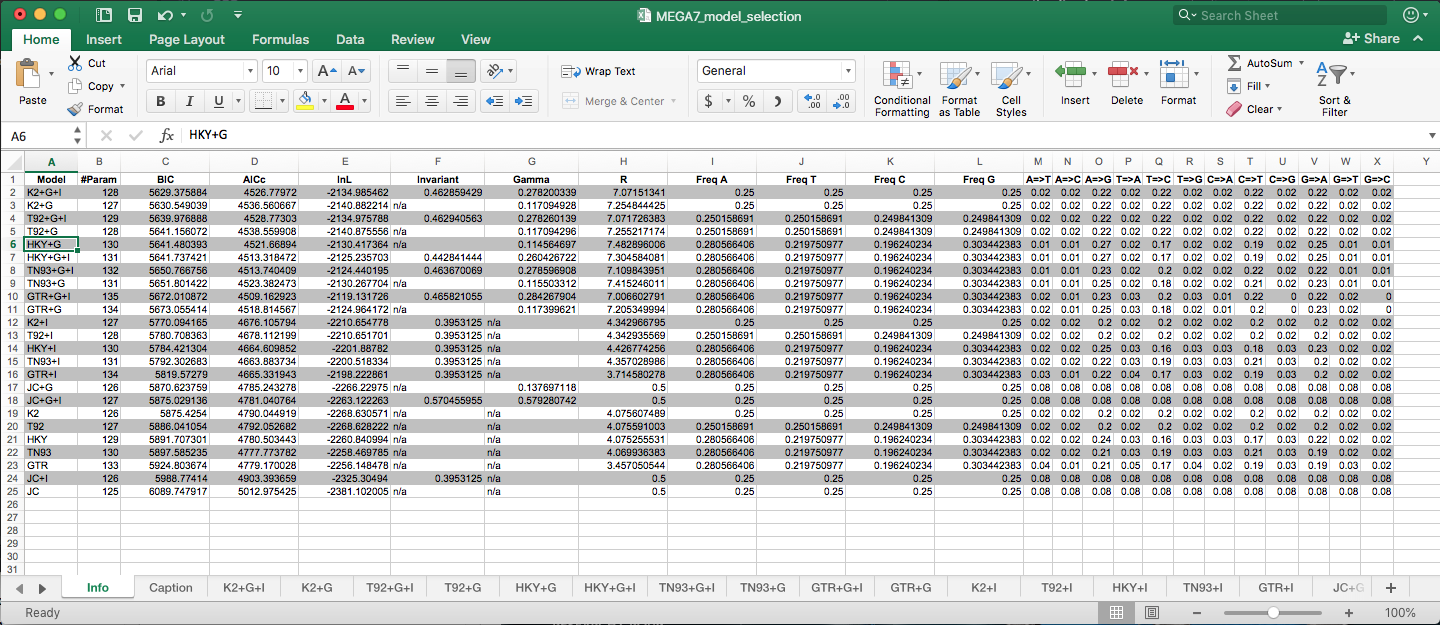# Phylogenetic models

genomics
phylogenetics

Notes on models for phylogenetics

Author

Siobhon Egan

Published

February 13, 2021

Phylogenetics - overview of model types

An excellent summary of the different models (listed below) is directly sourced from Evolution and Geonomics Workshop (link here).

Highly recommend you check out the rest of the website for some great resources and detailed information & tutorials.

The use of maximum likelihood (ML) algorithms in developing phylogenetic hypotheses requires a model of evolution. The frequently used General Time Reversible (GTR) family of nested models encompasses 64 models with different combinations of parameters for DNA site substitution. The models are listed here from the least complex to the most parameter rich.

Some common programs to carry out phylogenetic analysis include:

The below also include the settings if the model is available in these programs. Some good info here.

Jukes-Cantor (JC/JC69) equal base frequencies, all substitutions equally likely (Jukes and Cantor 1969)

• MrBayes `nst=1`
• PAUP `aaaaaa`
• PAML `aaaaaa`
• BEAST
• PhyML
• IQ-TREE

Felsenstein 1981 (F81) variable base frequencies, all substitutions equally likely (Felsenstein 1981)

• MrBayes `nst=1`
• PAUP `aaaaaa`
• PAML `aaaaaa`
• BEAST (check)
• PhyML
• IQ-TREE

Kimura 2-parameter (K80/K2) equal base frequencies, one transition rate and one transversion rate (Kimura 1980)

• MrBayes `nst=2`
• PAUP `abaaba`
• PAML `abbbba`
• BEAST
• PhyML
• IQ-TREE

Hasegawa-Kishino-Yano (HKY) variable base frequencies, one transition rate and one transversion rate (Hasegawa et. al. 1985). Note this model is very similar to K80 however allows for variable base frequencies. It is also commonly the default model in many programs.

• MrBayes `nst=2`
• PAUP `abaaba`
• PAML `abbbba`
• BEAST
• PhyML
• IQ-TREE

Tamura-Nei (TrN/TN93) variable base frequencies, equal transversion rates, variable transition rates (Tamura Nei 1993)

• MrBayes
• PAUP `abaaea`
• PAML `abbbbf`
• BEAST
• PhyML
• IQ-TREE

Kimura 3-parameter (K3P) variable base frequencies, equal transition rates, two transversion rates (Kimura 1981)

• MrBayes
• PAUP (`abccba`)
• PAML (`abccba`)
• BEAST
• PhyML
• IQ-TREE

Transition model (TIM) variable base frequencies, variable transition rates, two transversion rates

• MrBayes
• PAUP `abccea`
• PAML `abccbe`
• BEAST
• RAxML (check)
• PhyML
• IQ-TREE

Transversion model (TVM) variable base frequencies, variable transversion rates, transition rates equal

• MrBayes
• PAUP (`abcdbe`)
• PAML (`abcdea`)
• BEAST
• PhyML
• IQ-TREE

Symmetrical model (SYM) equal base frequencies, symmetrical substitution matrix (A to T = T to A) (Zharkikh 1994)

• MrBayes `nst=6`
• PAUP `abcdef`
• PAML `abcdef`
• BEAST
• PhyML
• IQ-TREE

general time reversible (GTR) variable base frequencies, symmetrical substitution matrix (e.g., Lanave et al. 1984, Tavare 1986, Rodriguez et. al. 1990)

• MrBayes `nst=6`
• PAUP `abcdef`
• PAML `abcdef`
• BEAST
• PhyML
• IQ-TREE

### Rate variation

In addition to models describing the rates of change from one nucleotide to another, there are models to describe rate variation among sites in a sequence. The following are the two most commonly used models and are generally available across all platforms and programs.

gamma distribution (G) gamma distributed rate variation among sites

proportion of invariable sites (I)* extent of static, unchanging sites in a dataset

# Programs

Some well resourced programs to carry out model selection include:

Depending on the program most will produce two sets of ‘scores’ to assess the models. the Akaike information criterion (AIC) and Bayesian information criterion (BIC). The lower the score the more support there is for a particular model. There are different reasons for choosing the best model based on the AIC or BIC scores. Generally the BIC score is used in most cases, however if in doubt its always best to do some more research to understand why you chose that value. Some references for more information on these criterion available here Luo A. et al. 2010; Sullivan J. and Joyce P. 2005,Brewer M. J. et al. 2016.

Example output from MEGA7 using Model Selection feature